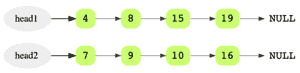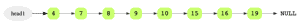# Merge Two Sorted Linked Lists

## Problem Statement

Given two sorted linked lists, merge them so that the resulting linked list is also sorted.

Consider two sorted linked lists as an example.The merged linked list should look like this:## Hint

• Use two iterators to scan both lists

## Try it yourself

typedef LinkedListNode* NodePtr;
//TODO: Write - Your - Code
}

## Solution

typedef LinkedListNode* NodePtr;

// if both lists are empty then merged list is also empty
// if one of the lists is empty then other is the merged list
} else if (head2 == nullptr) {
}

} else {
}

NodePtr temp = nullptr;
} else {
}

mergedTail->next = temp;
mergedTail = temp;
}

} else if (head2 != nullptr) {
}

}

void test(vector<int>& v1, vector<int>& v2, vector<int>& expected) {

}

int main(int argc, char* argv[]) {

vector<int> v1 = {1, 3, 5, 6};
vector<int> v2 = {2, 4, 6, 20, 34};
vector<int> expected = {1, 2, 3, 4, 5, 6, 6, 20, 34};

test(v1, v2, expected);

v1 = {1, 3, 5, 6};
v2 = {};
expected = {1, 3, 5, 6};

test(v1, v2, expected);

v1 = {1, 3, 5, 6};
v2 = {2, 4, 6, 20};
expected = {1, 2, 3, 4, 5, 6, 6, 20};

test(v1, v2, expected);
v1 = {4, 4};
v2 = {4, 4, 4};
expected = {4, 4, 4, 4 ,4};

test(v1, v2, expected);
}

## Solution Explanation

### Runtime Complexity

Linear, O(m + n) where m and n are lengths of both linked lists.

Constant, O(1)

### Solution Breakdown

Maintain a head and a tail pointer on the merged linked list. Then choose the head of the merged linked list by comparing the first node of both linked lists. For all subsequent nodes in both lists, you choose the smaller current node and link it to the tail of the merged list, and moving the current pointer of that list one step forward.

You keep doing this while there are some remaining elements in both the lists. If there are still some elements in only one of the lists, you link this remaining list to the tail of the merged list.

Initially, the merged linked list is NULL.

Compare the value of the first two nodes and make the node with the smaller value the head node of the merged linked list. In this example, it is 4 from head1.

Since it’s the first and only node in the merged list, it will also be the tail.

Then move head1 one step forward.

Learn in-demand tech skills in half the time